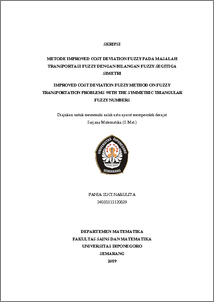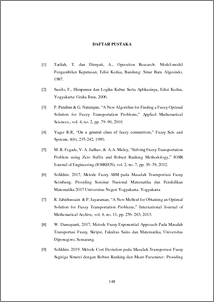# IMPROVED COST DEVIATION FUZZY METHOD ON FUZZY TRANSPORTATION PROBLEMS WITH THE SYMMETRIC TRIANGULAR FUZZY NUMBERS

Narulita, Fania Suci (2019) IMPROVED COST DEVIATION FUZZY METHOD ON FUZZY TRANSPORTATION PROBLEMS WITH THE SYMMETRIC TRIANGULAR FUZZY NUMBERS. Undergraduate thesis, UNDIP.Preview
PDF
218KbPDF
Restricted to Repository staff only

1439KbPreview
PDF
104Kb

## Abstract

A fuzzy transportation problem is the expansion of a crips transportation problem with the parameter of availability, request, transportation cost, or decision variable in the form of fuzzy number with the purpose of minimizing cost or maximizing profit. One of the methods to directly solve fuzzy transportation problem is the method of Cost Deviation Fuzzy. This method is able to give an optimal solution without having to look for the initial feasible solution of a fuzzy transportation problem. However, this method does not always give an optimal solution to a fuzzy transportation problem that is not balanced. This thesis will discuss the method of Improved Cost Deviation Fuzzy as an improvement of the method of Cost Deviation Fuzzy in order to solve a fuzzy transportation problem in which its parameter is symmetrical triangular fuzzy number dan to show the optimality of the method of Improved Cost Deviation Fuzzy. The method of Improved Cost Deviation Fuzzy is an optimal solution to either the balanced or not balanced fuzzy transportation problem. Keywords : Fuzzy Transportation Problem, Symmetric Triangular Fuzzy Number, Cost Deviation Fuzzy Method, Improved Cost Deviation Fuzzy Method.

Item Type: Thesis (Undergraduate) Q Science > QA Mathematics Faculty of Science and Mathematics > Department of Mathematics 84226 INVALID USER 11 Jun 2022 19:29 11 Jun 2022 19:29

Repository Staff Only: item control page